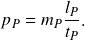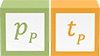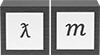# Mass-momentum symmetry

Mass-momentum symmetry is an alternative expression of length-mass symmetry but using traditional unit dimensions of momentum. Adding a superfluous time dimension describes the same physical phenomenon in dimensions LMT-1. Time is unnecessary because momentum quantifies a particle’s spatial attribute and not its rate of displacement (see wavelength operator)

The following illustration represents the mass-momentum symmetry in the three unit dimensions of momentum

The symmetry applies a single operator—the wavelength operator—to all three unit dimensions, producing observable quantities of wavelength and momentum in particles with and without rest mass. The time observable 𝑇𝜆 has no physical meaning but has historical and computational significance in using a system’s rest mass and velocity to find the average wavelength.

The equivalence of inertial mass and momentum becomes evident when we adopt a consistent definition of momentum for particles with and without rest mass.

## Derivation

In traditional unit dimensions, mass-momentum symmetry can be expressed from the definition of momentum. At the Planck scale, momentum is defined asRestating the definition of Planck momentum as an equality givesSince length-mass symmetry conserves wavelength and inertial mass on the left-hand side of the formula, the product of momentum and time is also conserved. 𝑝𝑃𝑡𝑃, and every combination of 𝑝𝑇𝜆 yields the quantum constant, 3.52 x 10-43 kgm.

ƛ𝑚 = 𝑝𝑇𝜆

### Potential### Invariant### Conserved quantity

3.52 x 10-43 kgm

3.52 x 10-43 kgm

The mass-momentum symmetry is reflected in non-relativistic quantities of particle wavelength and inertial mass, demonstrated in the following table using mechanical properties of photons. The table applies the wavelength operator 𝑙𝑃 / ƛ to the Planck length, mass, and momentum, producing observable quantities of wavelength, inertial mass, and momentum proportional to the Planck scale. Quantities calculated using New Foundation Model operators are equivalent to historical formulas in which Planck’s constant is used.

** The table shows the length form of the wavelength operator but mass and time forms can also be used

** Natural formulas shown in the table are the same for particles with and without rest mass

Mass-momentum symmetry